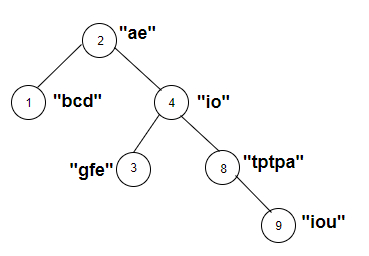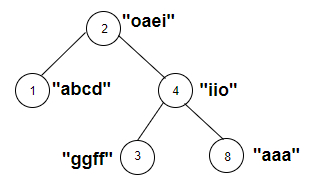# Count the nodes of the tree whose weighted string contains a vowel in C++

Given a binary tree with weights of its nodes as strings. The goal is to find the number of nodes that have weights such that the string contains a vowel. If weight is ‘aer’ then it has vowels ‘a’ and ‘e’ so the node will be counted.

For Example

## Input

The tree which will be created after inputting the values is given below −## Output

Count the nodes of the tree whose weighted string contains a vowel are: 5

## Explanation

we are given with the tree nodes and the string weights associated with each node. Now we check whether the string of nodes contains vowels or not.

NodeWeightvowelsyes/no
2aeeyes
1bcdNo vowelno
4ioi,oyes
3gfeeyes
8tptpaayes
9ioui,o,uyes

## Input

The tree which will be created after inputting the values is given below −## Output

Count the nodes of the tree whose weighted string contains a vowel are: 3

## Explanation

with the tree nodes and the string weights associated with each node. Now we check whether the string of nodes contains vowels or not.
NodeWeightvowelsyes/no
2oaeio,a,e,iyes
1abcdNo vowelno
4iioi,oyes
3ggffNo vowelno
8aaaayes

Approach used in the below program is as follows

In this approach we will apply DFS on the graph of the tree to traverse it and check if the weight of the node has a string containing a vowel. Take two vectors Node_Weight(100) and edge_graph for this purpose.

• Initialize Node_Weight[] with the weights of nodes.

• Create a tree using vector edge_graph.

• Take a global variable vowel and initialize it with 0.

• Function check(string check_it) takes s string and returns true if check_it contains a vowel in it.

• Take length = check_it.length() as number of characters in check_it.

• Traverse check_it using for loop from index i=0 to i<length.

• Take each check_it[i] as converted to lowercase and stored in c.

• If c is equal to either of the vowels ( ‘a’, ‘e’ ‘i’, ‘o’, ‘u’ ) then return true else return false.

• Function string_vowel(int node, int root) takes a node and root node of a tree and returns the count of nodes in the given tree whose weight contains a vowel as character.

• Take str = Node_Weight[node].

• If check(str) returns true then increment the vowel.

• Traverse tree in vector edge_graph[node] using for loop.

• Call string_vowel(it, node) for the next node in the vector.

• At the end of all functions we will have a vowel as the number of nodes with weights having vowels in it.

## Example

Live Demo

#include <bits/stdc++.h>
using namespace std;
vector<string> Node_Weight(100);
vector<int> edge_graph;
int vowel = 0;
bool check(string check_it){
int length = check_it.length();
for(int i = 0; i <length; i++){
char c = tolower(check_it[i]);
if(c == 'a' ||c == 'e' ||c == 'i' ||c == 'o' ||c == 'u'){
return true;
}
}
return false;
}
void string_vowel(int node, int root){
string str = Node_Weight[node];
if(check(str)){
vowel++;
}
for (int it : edge_graph[node]){
if(it == root){
continue;
}
string_vowel(it, node);
}
}
int main(){
//weight of the nodes
Node_Weight = "ae";
Node_Weight = "bcd";
Node_Weight = "io";
Node_Weight = "gfe";
Node_Weight = "tptpa";
Node_Weight = "iou";
//create graph edge
edge_graph.push_back(1);
edge_graph.push_back(4);
edge_graph.push_back(3);
edge_graph.push_back(8);
edge_graph.push_back(9);
string_vowel(2, 2);
cout<<"Count the nodes of the tree whose weighted string contains a vowel are: "<<vowel;
return 0;
}

## Output

If we run the above code it will generate the following output −

Count the nodes of the tree whose weighted string contains a vowel are: 5